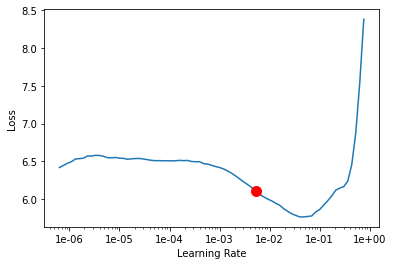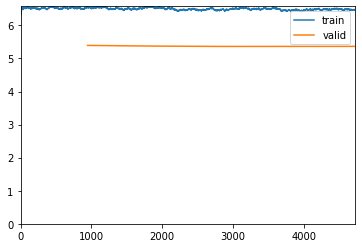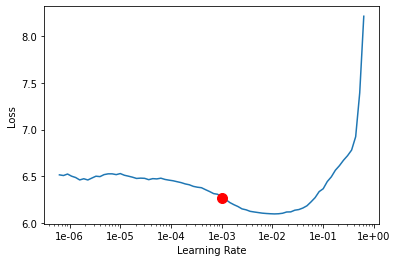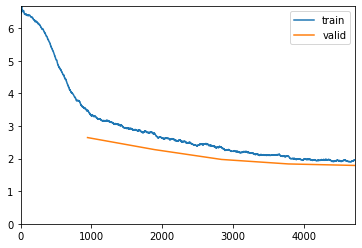# Get_transforms() reduced my accuracy

Hi.

I am working on a classifier using the Food-101 dataset. I am trying to react a SOTA score of >=90% using ResNet50. Initially, I was sitting at 75% accuracy. When I included label smoothing, I jumped to a sweet 81% but now when I use fastai’s `get_transforms`, I dropped to 79%. I also noticed that even though I’m using image augmentation, there wasn’t an increase in my data’s dimensions when I was running the epoch.

Currently, the `tfms` variable is my augmentation:

``````np.random.seed(42)

path = '/content/food-101/images/train'
file_parse = r'/([^/]+)_\d+\.(png|jpg|jpeg)\$'
tfms = get_transforms(do_flip=True,flip_vert=True, max_rotate=10.0, max_zoom=1.1, max_lighting=0.2, max_warp=0.2, p_affine=0.75, p_lighting=0.75)

data = ImageList.from_folder(path).split_by_rand_pct(valid_pct=0.2).label_from_re(pat=file_parse).transform(tfms, size=224).databunch()

top_1 = partial(top_k_accuracy, k=1)
learn = cnn_learner(data, models.resnet50, metrics=[accuracy, top_1], loss_func = LabelSmoothingCrossEntropy(), callback_fns=ShowGraph)

learn.lr_find()
learn.recorder.plot(suggestion=True)
``````My learning rate also slightly increased from 1.02e-06 to 1.023e-06 when I applied `get_transforms()` to my model.

I’m running another 5 epochs. The accuracy is low due to label smoothing. This isn’t an issue:

``````learn.fit_one_cycle(5, max_lr=slice(1.023e-06/5, 1.023e-06/15))
learn.save('stage-2')
``````
epoch train_loss valid_loss accuracy top_k_accuracy time
0 6.549849 5.393574 0.009835 0.009835 18:39
1 6.520850 5.373156 0.009835 0.009835 17:57
2 6.468420 5.362789 0.009901 0.009901 17:49
3 6.461528 5.363743 0.009769 0.009769 17:51
4 6.473554 5.364657 0.009901 0.009901 18:05Now I’m pulling out another learning rate. It jumped to 1.03e-06.

``````learn.unfreeze()
learn.lr_find()
learn.recorder.plot(suggestion=True)
``````Running another 5 epochs using the new LR and saving it. In this round, the label smoothing does not seem to have the same effect as it did on the earlier run. The model is now showing better accuracy than the earlier 5 epochs:

``````learn.fit_one_cycle(5, max_lr=slice(1e-03/5, 1e-03/15))
learn.save('stage-3')
``````
epoch train_loss valid_loss accuracy top_k_accuracy time
0 3.476312 2.645357 0.491683 0.491683 18:11
1 2.781384 2.276255 0.599670 0.599670 18:22
2 2.356208 1.974409 0.677426 0.677426 18:31
3 2.068619 1.836324 0.732409 0.732409 18:26
4 1.943876 1.789893 0.742310 0.742310 18:20When I was checking the confusions using this block of code:

``````interp.plot_confusion_matrix()
interp.most_confused(min_val=5)
interp.plot_multi_top_losses()
interp.plot_confusion_matrix(figsize=(20, 20), dpi=200)
``````

I got this output about my validation set:

`15081 misclassified samples over 15150 samples in the validation set.`

Finally, when I validate the model with the test set, this is what I get:

``````path = '/content/food-101/images'
data_test = ImageList.from_folder(path).split_by_folder(train='train', valid='test').label_from_re(file_parse).transform(size=224).databunch()

`[1.602944, tensor(0.7987), tensor(0.7987)]`